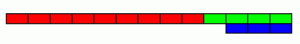Using 3 rods of lengths from 1 to 10 units, and not using any rod more than once, you can measure all the lengths in whole units from 1 to 10 units.

For example with rods of lengths 3, 4, and 9 the measurements are:

4 – 3 = 1;   9 – 4 – 3 = 2;   3;   4;   9 – 4 = 5;   9 – 3 = 6;   3 + 4 = 7;   9 + 3 – 4 = 8;   9 and 9 + 4 – 3 = 10 (as illustrated).How many ways can you find to do all these measurements with 3 rods?

Using 3 rods of ANY integer lengths, what is the greatest length N for which you can measure all lengths from 1 to N units inclusive? Can you beat 10 units?

What is the greatest length that can be measured using 4 of the rods in this way?